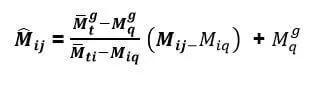# GATE Normalization Process | How GATE Score is Calculated?

The Graduate Aptitude Test in Engineering (GATE) is one of the topmost taken up entrance exams by graduates. This is a national level entrance exam taken up by Engineering graduates to enter into various streams like PG courses, government jobs, PSUs, and many more. As the GATE 2019 examination is completed successfully and the GATE result are released, candidates are waiting to know their GATE score. There is some time for the GATE board to release candidates scores. In the meantime, many of you might be wondering how is this GATE score calculated? What is the GATE Normalization Process and how is it done? Well, we will answer all your queries. Read the blog completely to know more about the Normalization process of GATE Exam 2019.

## GATE Normalization Process

The Normalization Process is a process used for converting raw marks into scores. After the evaluation of GATE Answers, the raw marks i.e., actual marks obtained in GATE will be considered for calculating the GATE Score based on qualifying marks.

Take Up GATE Online Tests Here

As GATE is one of the major national level exams, lakhs of candidates appear every year and it is not possible to conduct the exam in one go. So, GATE Exam is conducted in different sessions. Hence, it is normal that there would be variations in the difficulty level of the exam. To resolve the difference in the difficulty level of the GATE exam, the normalization process is applied. For the papers which are conducted in multiple session, this normalization process is applied. The subjects conducted in multiple sessions are

• Computer Science (CS)
• Civil Engineering (CE)
• Electrical Engineering (EE)
• Electronic & Communication Engineering (EC)
• Mechanical Engineering (ME)

Based on various parameters and various GATE normalization Process, the committee of GATE has come up with a formula for calculating the normalized marks for the multiple session papers.

Normalization mark of Jth candidate in the ith session ijwhere,

Mij– is the actual marks secured by jth candidates in the ith sessions

g– is the average marks of the top 0.1% of the candidates considering all sessions

Mgq – is the sum of mean and standard deviation marks of the candidates in the paper considering all sessions

ti – is the average marks of the top 0.1% of the candidates in the ith sessions

Miq – is the sum of the mean marks and standard deviation of the ith session

Below is the graph that helps you to understand the relationship between the two scores better.The above graph shows the linear relationship between “actual marks” and “normalized marks” of a candidate, in multiple session subject.

After the answers are checked and assessed, the normalized marks through GATE Normalization Process of a candidate will be calculated corresponding to the actual marks secured by the candidate in the examination. The GATE 2019 Scorecard will be released based on the GATE normalized marks. The actual marks secured by the candidate will be used for calculating the GATE 2019 score for the papers which are conducted in a single session only.

## Calculation of GATE Score for all Papers

After the normalization of the marks, the final GATE Score 2019 will be calculated using the below formulawhere,

M – marks secured by the candidate in the individual papers (actual marks for single session papers and normalized marks for multi-session papers)

Mq – is the qualifying marks for general category candidate in the paper

M̅t  – is the mean of marks of top 0.1% or top 10 (whichever is larger) of the candidates who appeared in the paper (in case of multi-session papers including all sessions)

S– 350, is the score assigned to Mq

S– 350, is the score assigned to t

In the GATE 2019 formula, Mq is normally 25 marks (out of 100) or +σ, whichever is larger. In this case, μ is the mean and σ is the standard deviation of marks of all the candidates who appeared in the paper.

Thus, with this GATE Normalization Process, the GATE score of all candidates is determined. candidates will be posted when the GATE score is released. Stay tuned for more information and check out our latest GATE Preparation Books available online.

[related_books catid=”242″ product_type=”ebook” algo=”Latest”]